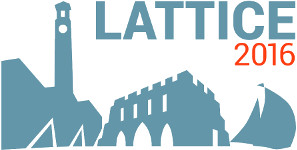#The 34th International Symposium on Lattice Field Theory (Lattice 2016)

Jul 24 – 30, 2016
Highfield Campus, University of Southampton
Europe/London timezone

## Lambda-Nucleon and Sigma-Nucleon interactions from lattice QCD with physical masses

Jul 29, 2016, 3:00 PM
20m
Building B2a Room 2065 (Highfield Campus, University of Southampton)

### Speaker

Dr Hidekatsu Nemura (Center for Computational Sciences, University of Tsukuba)

### Description

We present our recent study on baryon-baryon (BB) interactions from lattice QCD with almost physical quark masses corresponding to $(m_\pi,m_K)\approx(146,525)$ MeV and large volume $(La)^4=(96a)^4\approx$ (8.2 fm)$^4$. In order to make better use of large scale computer resources, a large number of BB interactions from NN to $\Xi\Xi$ are calculated simultaneously. In this contribution, we focus on the strangeness $S=-1$ channels of the hyperon interactions by means of HAL QCD method. More recent results will be presented which include $\Lambda$N$-\Sigma$N coupled-channel potential comprising the tensor force as well as increasing the Monte Carlo samples than shown in the past. References:  H. Nemura, et al., arXiv:1604.08346 [hep-lat]. [enter link description here] : https://arxiv.org/abs/1604.08346

### Primary author

Dr Hidekatsu Nemura (Center for Computational Sciences, University of Tsukuba)

 Slides